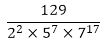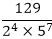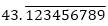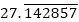# RD Sharma Class 10 Ex 1.6 Solutions Chapter 1 Real Numbers

In this chapter, we provide RD Sharma Class 10 Ex 1.6 Solutions Chapter 1 Real Numbers for English medium students, Which will very helpful for every student in their exams. Students can download the latest RD Sharma Class 10 Ex 1.6 Solutions Chapter 1 Real Numbers pdf, Now you will get step by step solution to each question.

# Chapter 1: Real Numbers Exercise – 1.6

### Question: 1

Without actually performing the long division, state whether the following rational numbers will have a terminating decimal expansion or a non-terminating repeating decimal expansion.

(i)  23/8

(ii) 125/441

(iii) 35/50

(iv) 77/210

### Solution:

(i) The given number is 23/8

Here, 8 = 23 and 2 is not a factor of 23.

So, the given number is in its simplest form.

Now, 8 = 23 is of the form 2m × 5n, where m = 3 and n = 0.

So, the given number has a terminating decimal expansion.

(ii) The given number is 125/441

Here, 441 = 32 × 72 and none of 3 and 7 is a factor of 125.

So, the given number is in its simplest form.

Now, 441 = 32 × 72 is not of the form 2m × 5n

So, the given number has a non-terminating repeating decimal expansion.

(iii) The given number is 35/50 and HCF (35, 50) = 5.

Here, 7/10 is in its simplest form.

Now, 10 = 2 × 5 is of the form 2m × 5n, where in = 1 and n = 1.

So, the given number has a terminating decimal expansion.

(iv) The given number is 77/210 and HCF (77, 210) = 7.

Here, 11/30 is in its simplest form. 30

Now, 30 = 2 × 3 × 5 is not of the form 2m × 5n.

So, the given number has a non-terminating repeating decimal expansion.

(v) The given number isClearly, none of 2, 5 and 7 is a factor of 129.

So, the given number is in its simplest form.

### Question: 2

Write down the decimal expansions of the following rational numbers by writing their denominators in the form of 2m × 5n, where m, and n, are the non- negative integers.

(i)  3/8

(ii)  13/125

(iii)  7/80

(iv) 14588/625

### Solution:

(i) The given number is 3/8

Clearly, 8 = 23 is of the form 2m × 5n, where m = 3 and n = 0.

So, the given number has terminating decimal expansion.

(ii) The given number is 13/125

Clearly, 125 = 53 is of the form 2m × 5″, where m = 0 and n = 3.

So, the given number has terminating decimal expansion.

(iii) The given number is 7/80.

Clearly, 80 = 24 × 5 is of the form 2m × 5n, where m = 4 and n = 1.

So, the given number has terminating decimal expansion.

(iv) The given number is 14588/625

Clearly, 625 = 54 is of the form 2m × 5n, where m = 0 and n = 4.

So, the given number has terminating decimal expansion?

(v) The given number isClearly, 22 × 57 is of the form 2m × 5n, where in = 2 and n = 7.

So, the given number has terminating decimal expansion?

### Question: 3

What can you say about the prime factorization of the denominators of the following rational?

(i) 43.123456789

(iv) 0.120120012000120000

### Solution:

(i) Since 43.123456789 has terminating decimal expansion. So, its denominator is of the form 2m × 5n, where m, n are non-negative integers.

(ii) Sincehas non-terminating decimal expansion. So, its denominator has factors other than 2 or 5.

(iii) Sincehas non-terminating decimal expansion. So, its denominator has factors other than 2 or 5.

(iv) Since 0.120120012000120000 … has non-terminating decimal expansion. So, its denominator has factors other than 2 or 5.

All Chapter RD Sharma Solutions For Class10 Maths

I think you got complete solutions for this chapter. If You have any queries regarding this chapter, please comment on the below section our subject teacher will answer you. We tried our best to give complete solutions so you got good marks in your exam.